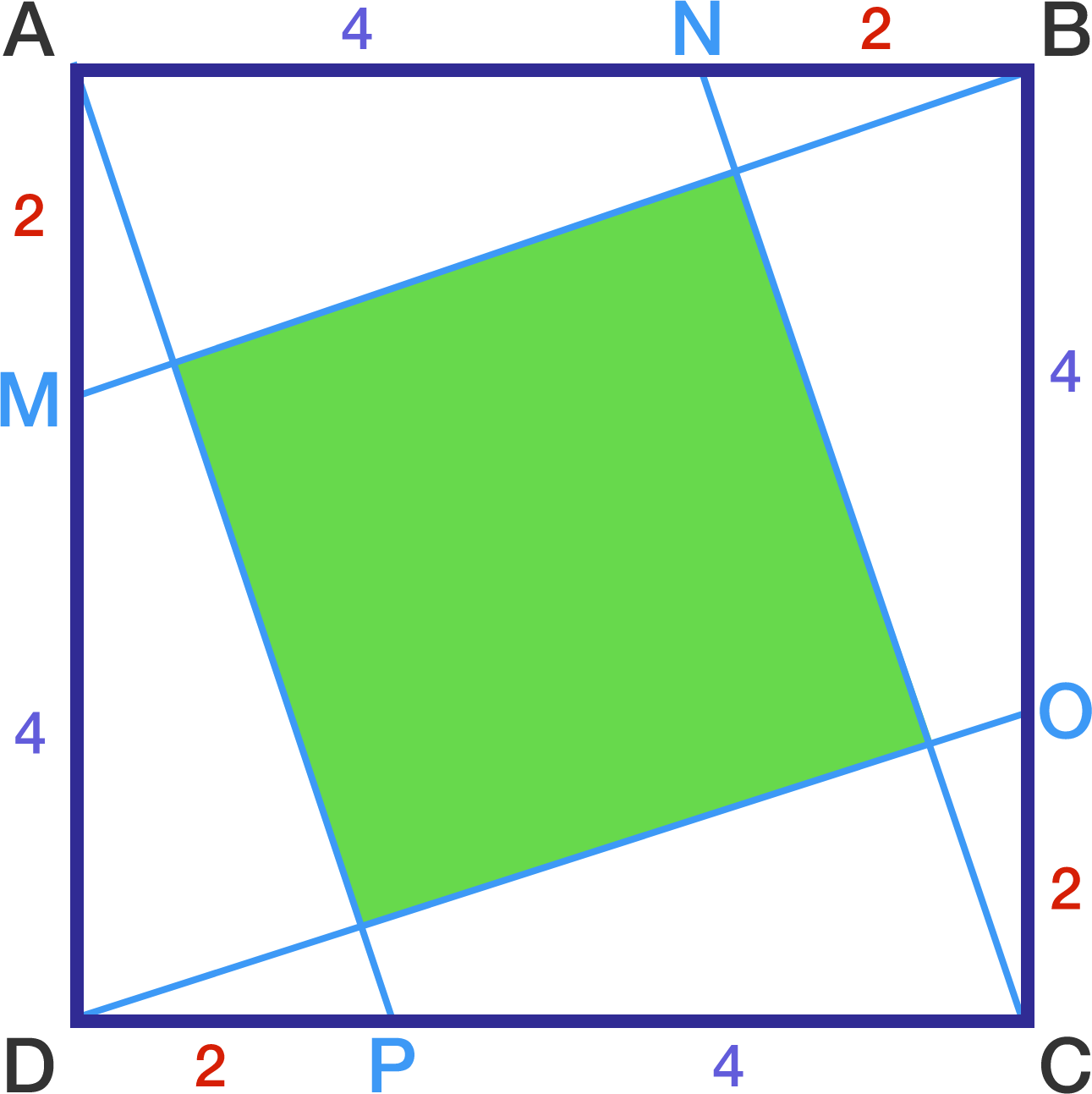# Slanted tiles

Geometry Level 2The above shows a square $ABCD$ with side length 6.

$M,N,O,P$ are points on the sides $AD,BA,CB,DC$, respectively such that $AM = DP = CO = BN = 2$.

Find the area of the green square.

×